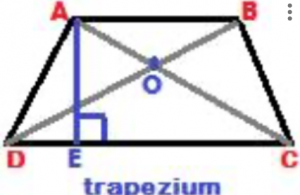Before we derive the area of a trapezoid, let us first get familiar with a few basic terms in a trapezoid. A trapezoid (or trapezium) is a quadrilateral in which one pair of sides is parallel. The parallel sides are called the bases of a trapezoid. The other two sides, which are not parallel to each other, are called the lateral sides of a trapezoid.If the lateral sides are equal, then the trapezoid is called an isosceles trapezoid. In the above figure, ABCD is a trapezoid. The two sides AB and DC are parallel to each other, i.e. AB // DC. The two sides AB and DC are the bases of the trapezoid ABCD. And, the other two sides AD and BC, which are not parallel to each other, are called the lateral sides of the trapezoid ABCD. (The trapezoid is called anisosceles trapezoid, if the two lateral sides are equal in length).

Area of a trapezoid:

In the above trapezium, ABCD, AB and DC are the two bases of the trapezium. The line AE is perpendicular to both the bases AB and DC. Now, in triangle ABD, if AB is base, then AE is height; and, in triangle BCD, if DC is the base, then the height is again AE.

So, the areas of the two triangles ABD and BCD are:

• Area of triangle ABD = ½ × (AB) × AE,
• Area of triangle BCD = ½ × (DC) × AE

Therefore, area of a trapezoid ABCD = sum of the areas of the two triangles ABD and BCD, i.e.

Area of a trapezoid ABCD = ½ × (AB + DC) × AE

This formula can be written in a more general way, expressing the area of a trapezoid as: ½ × (sum of the parallel sides) × (distance between the parallel sides)